# Fixed versus random-effects meta-analysis – efficiency and confidence interval coverage

Meta-analysis is a critical tool for synthesizing existing evidence. It is commonly used within medical and clinical settings to evaluate the existing evidence regarding the effect of a treatment or exposure on an outcome of interest. The essential idea is that the estimates of the effect of interest from previous study are pooled together. A choice which has to be made when conducting a meta-analysis is between fixed-effects and random-effects. In this post we’ll look at some of the consequences of this choice, when in truth the studies are measuring different effects.

Setup
We’ll assume that we have estimates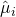from n studies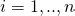of our effect of interest. Examples of possible effect measures are a difference between means between two treatment groups or a log odds ratio from logistic regression fits comparing to exposure groups. Along with the estimated effect, we also need the estimated variance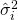or standard error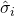.

Fixed-effects meta-analysis
In a fixed-effects meta-analysis, we assume that each of the studies included are estimating the same underlying parameter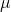. In some settings this assumption might be plausible – for example if the studies have all been conducted in the same population, they have used the same inclusion criteria, the treatments have been given in the same way, and outcomes have been measured consistently. In the fixed-effects approach, the different effect estimates are attributed purely to random sampling error.

Random-effects meta-analysis
In contrast, in a random-effects meta-analysis, we assume that each study is estimating a study-specific true effect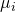(note the lack of a hat here – these are the true effects, not the estimated effects). Interest then lies in estimating the mean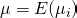and variance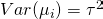of these true effect sizes across the population of potential studies. In a random-effects meta-analysis, the observed heterogeneity in the estimatesis attributed to two sources: 1) between-study heterogeneity in true effects, and 2) within-study sampling error.

Fixed versus random-effects meta-analysis
Which approach we use affects both the estimated overall effect we obtain and its corresponding 95% confidence interval, and so it is important to decide which is appropriate to use in any given situation. My personal view is that this decision ought to be made on the basis of knowledge about the constituent studies, rather than on the basis of actually looking at the point estimates (i.e. before you see the data).

In a fixed-effects analysis, the study estimates are weighted purely according to their estimated variances. If there is one very large contributing study, the small studies will be given very little weight. This is because under an assumption of common effects, this weighting results in the most precise estimate of the common effect.

Conversely, in a random-effects meta-analysis, the weights are also a function of the estimated value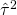. When this estimated variability in true effects is large, smaller studies are given proportionately more weight than they are in the fixed-effects meta-analysis. This is because, if there is true heterogeneity in effects, if studyis very large, it gives a very precise estimate of, the study specific effect. Of course this does give information about the parameter, but because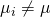, it gives less information than when a fixed-effects analysis is assumed.

A further obvious difference between the two approaches is that the calculated standard error is smaller from a fixed effects meta-analysis than that from a random-effects meta-analysis. This may lead researchers to believe the the fixed-effects estimate is more precise. In the remainder of the post we’ll conduct a small simulation study, and see that this doesn’t hold if in truth there is heterogeneity in the effects between estimated by the studies. We’ll also see that the confidence intervals from the fixed effects analysis may have coverage which is substantially lower than the 95% value, when there is between study heterogeneity in true effects, i.e. when the random-effects approach is correct.

A simulation study in R
To perform our simulation study, we will simulate repeated meta-analyses of 30 studies. For each study, we simulate a true effect from a super-population distribution of true effects which is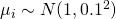. We then simulate within-study SDs (from a gamma distribution), and lastly simulate the observed effect estimates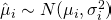. To be clear, we are simulating data consistent with the random-effects meta-analysis approach, so we should expect this analysis method to ‘perform well’.

We then perform both a fixed-effects and random-effects meta-analysis. To do this we will make use of the mvmeta package, written by my colleague Antonio Gasparrini. For the random-effects meta-analysis we will use the traditional DerSimonian and Laird moment based approach. The code is shown below:

library(mvmeta)
nStudies <- 30
nSims <- 1000

fixedEffectEsts <- array(0, dim=c(nSims))
fixedCI <- array(0, dim=c(nSims,2))
randomEffectEsts <- array(0, dim=c(nSims))
randomCI <- array(0, dim=c(nSims,2))

for (i in 1:nSims) {
print(i)
#sample nStudies true effects from population
trueEffects <- rnorm(nStudies, mean=1, sd=0.1)

#sample within study standard deviations
withinStudySD <- rgamma(nStudies, shape=2.5, scale=0.04)

#sample estimate from study
studyEstimate <- rnorm(nStudies, mean=trueEffects, sd=withinStudySD)

#fixed effects meta-analysis
maFixed <- mvmeta(studyEstimate~1, S=withinStudySD^2, method="fixed")
fixedEffectEsts[i] <- coef(maFixed)
fixedCI[i,] <- c(coef(maFixed)-1.96*maFixed$vcov^0.5,coef(maFixed)+1.96*maFixed$vcov^0.5)

#random-effects meta-analysis
maRandom <- mvmeta(studyEstimate~1, S=withinStudySD^2, method="mm")
randomEffectEsts[i] <- coef(maRandom)
randomCI[i,] <- c(coef(maRandom)-1.96*maRandom$vcov^0.5,coef(maRandom)+1.96*maRandom$vcov^0.5)
}

mean(fixedEffectEsts)
sd(fixedEffectEsts)

mean(randomEffectEsts)
sd(randomEffectEsts)

#ci coverage
mean((fixedCI[,1]<1) & (fixedCI[,2]>1))
mean((randomCI[,1]<1) & (randomCI[,2]>1))


The last lines of R code calculate the mean and SD of the fixed and random-effects estimates across the 1,000 simulations, and then the coverage of the 95% confidence intervals. When I ran the code I obtained:

> mean(fixedEffectEsts)
 0.9990649
> sd(fixedEffectEsts)
 0.04939415
>
> mean(randomEffectEsts)
 1.000967
> sd(randomEffectEsts)
 0.0242558
>
> #ci coverage
> mean((fixedCI[,1]<1) & (fixedCI[,2]>1))
 0.322
> mean((randomCI[,1]<1) & (randomCI[,2]>1))
 0.926


The first thing to notice is that the fixed-effects approach is still unbiased, even though the data are being simulated based on a random-effects model. However, we see that the SD is much larger for the fixed-effects approach (0.049 vs 0.024 for the random-effects). Or put another way, the random-effects estimator is a more precise estimator (when there is in between study heterogeneity in true effects). This is because, as described previously, the fixed-effects gives large studies more weight than is optimal, when there is between study heterogeneity in true effects.

This finding is important – although the standard error reported by a fixed effects meta-analysis is smaller than the random-effects meta-analysis, the random-effects estimate is actually likely to be closer to the parameter of interest. The explanation for this apparent contradiction is that the standard error calculated by the fixed effects approach is invalid when in truth there is between study heterogeneity. A clear manifestation of this can be seen in the coverage of the supposed 95% confidence intervals from the two approaches (the last part of the output). While the random-effects confidence interval included the true parameter value on 92.6% of the 1,000 simulations, the fixed-effects interval included it only 32.2% of the time – severe under coverage. This is a direct consequence of the biased standard error being used by the fixed-effects approach.

It is important to emphasize that the superior efficiency of the random-effects estimator and the under coverage of the fixed-effects 95% confidence interval have occurred here because we have simulated data under a random-effects assumption. If instead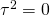in truth, the fixed-effects approach would perform better – its estimates would be more precise and confidence interval would have correct (or close to correct) coverage.

Conclusions
The conclusions I draw from this small simulation study is that one should be wary of using a fixed effects analysis unless one is fairly confident that the studies in the meta-analysis are estimating the same common effect. When, as I believe is often the case for a whole host of reasons, there will be between-study heterogeneity in true effects, a random-effects approach ought to be adopted – we will obtain a more precise estimate and the confidence interval will have the correct (or close to correct) coverage.

For more reading on this topic, I’d recommend looking at the very readable extensive article here.

### 10 thoughts on “Fixed versus random-effects meta-analysis – efficiency and confidence interval coverage”

1.I appreciate that you’re trying to demystify things, but the situation is not as clear cut as you describe.

Fixed effectS (plural) analysis doesn’t require that all the study effects are the same; it provides inference on an average effect, averaging over a population like the one in the studies at hand. See e.g. Hedges and Vevea 1998, or the Handbook of Meta Analysis & Evidence Synthesis. As shown in recent work by Lin and Zheng, the fixed effects approach – in very many situations – yields as efficient an estimate as one would get combining all the data together and performing a regression analysis that adjusted for study. The under-coverage you describe goes away if all the contributing studies are large, even if they are not homogeneous, and this situation will often hold in practice.

Use random effects analysis if you want to know about things that random effects analysis tell you, and fixed effects if you’re interested in what they can tell you. Neither need be “right” or “wrong” or even “better” or “worse”, they are just different.

•Thanks for your comment, but I’m afraid I don’t agree!

1) You say that a fixed effects approach doesn’t assume that the study effects are the same. From the Cochrane website – “Methods of fixed effect meta-analysis are based on the mathematical assumption that a single common (or ‘fixed’) effect underlies every study in the meta-analysis.”. Or, from a paper by Higgins et al published here: “A ‘fixed effect’ model assumes that a single parameter value is common to all studies, and a ‘random-effects’ model that parameters underlying studies follow some distribution.”. A final quote to the same effect, from a recent paper by Riley: “A fixed effect meta-analysis assumes all studies are estimating the same (fixed) treatment effect, whereas a random effects meta-analysis allows for differences in the treatment effect from study to study.” and later in the same paper “Use of a fixed effect meta-analysis model assumes all studies are estimating the same (common) treatment effect.”.

2) The paper you mention by Lin and Zheng I presume is this one. This is a nice paper, and the result (that often you can get the same estimate from a fixed effects meta-analysis as one where you fit a model to the original study data and include study as a fixed effect) is certainly of great interest, but I didn’t talk about analysing the original individual level data. They note that the fixed effects assumption does not affect the type 1 error rate, which is of course correct – if the effect is zero in every study, the fixed effects approach is appropriate. But as far as I can see they didn’t examine confidence interval coverage in the non-null case (which is what I looked at in the post).

3) You say that the undercoverage I describe will disappear if all the contributing studies are large. I don’t think this is the case – the fixed effects variance estimator is derived (and is valid) under an assumption that the studies are estimating a common effect – when this does not hold (as I assumed in my post), it ignores the contribution to variance from the between-study variability in effects. If the within-study variances are all very small (when all the contributing studies are large), the fixed effects standard error will be very close to zero, which does not correctly reflect the repeated sampling variability of the fixed effects estimator (when in truth, the random-effects model is true). To empirically verify this, I took the R code in the post, and changed the within-study SD line to read:
withinStudySD <- rep(0.000001,nStudies) which corresponds to all studies being very large. As I expected, the 95% confidence interval from the fixed effects analysis had low (0 in fact) coverage of the true mean effect. My intention was not to criticize the fixed effects approach per se. I made clear that in the simulations data were generated consistent with a random-effects assumption, and so one should not necessarily be surprised that the fixed effects approach encounters issues in this case. I didn't say one was right and one was wrong!

2.I think the big mistake in your simulation is assuming that the method you choose to analyze the data (random effect model) is also the mechanism through which the data is generated in a meta-analysis. Obviously, that is impossible because the studies in a meta-analysis represent the whole population and there is no possibility of a random selection from a fictitious normal distribution as you have done in the simulation. The result is that prediction is impossible. I would recommend you rerun the simulation and generate the study effects from N(mu,phi_i+sigma_i) where mu is the unknown common effect and phi_i and sigma_i are two sources of variance due to non-random and random error respectively. This is probably the data generation mechanism in meta-analysis and now you can see if smoothing and shrinkage (random effects) is really more efficient that fixed effect estimators.

•Thanks for your comment Suhail. You say that it is impossible for the random effects analysis to be correct, because “the studies in a meta-analysis represent the whole population and there is no possibility of a random selection from a fictitious normal distribution as you have done in the simulation”. I do not really understand why you say that the (true) effects of the studies analysed in the meta-analysis must necessarily represent the whole population. The key idea of a random-effects meta-analysis is that the true effects being measured by the included studies are assumed to have been (in some sense) sampled from a large population of true effects which might have occurred in a hypothetical universe of studies. This universe of studies would encompass the various differences in design, study population, etc, which cause the variability in true effects. One can argue (and maybe that is what you are saying) that this notion doesn’t make much sense because this universe of studies is ill defined. However, I did not argue in the post that the random effects model/analysis is necessarily correct, but merely examined its repeated sampling properties (and that of the fixed effects approach) when in truth data are indeed generated according to the setup assumed by the random-effects analysis.

Lastly, in light of the rest of your comment, I am confused by your suggestion to generate the “study effects from N(mu,phi_i+sigma_i)” distribution (I assume by effects you mean the actual observed study effect estimates) – if you were to do this, this would also correspond to a random effects analysis model, where the true between-study variance is phi_i and the common within-study variance is sigma_i.

3.Hi Jonathan, thanks for your response to my comment. The problem here is that the studies in a meta-analysis represent the *entire* universe (as we know it), and thus to say that the universe of studies is ill defined seems to me a gross under-statement. I would prefer to say that a separate universe of studies does not exist if the studies themselves make up this universe. Thus, given that these studies make up the whole population, each effect is of interest. Now, you could assume any model to pool these effects, I have no issue with that, but to take this a step further and assume that because you *choose* to analyze this way then this *must* be the mechanism for generation of the data is counter-intuitive. There is no *universe*, so how is this the mechanism of data generation? You are simply matching your simulation to what you use for analysis thereby creating performance measures around the estimator that you are more or less expecting to find.

This brings me to the next point – varying true effects. If each study had a completely different true effect, why would you even choose to meta-analyze? For example, if malaria prevalence in five countries were completely different why would I choose variance weights to pool these? I would rather standardize the prevalence across countries based on population weights thus achieving a standardized prevalence estimate for the region. Meta-analysis only makes sense if the underlying unknown effect has a value (mu) for the most common scenario (as opposed to a common underlying effect) and each study departs from this by random or systematic errors (inclusive in this systematic error is departure from the most common scenario). In this case your simulation should generate each study effect from N(mu, phi2_i+sigma2_i) where *both* phi2_i and sigma2_i are unique to the study under consideration. Phi2_i is not between study variance as it is not common to all studies.

While simulation generation of sigma2_i is straightforward, I can make a suggestion for phi2_i. Assume that study perfection, q_i, can be defined on a scale between asymptotic 0 and 1 for worst and best study respectively. Assume a fixed baseline phi2_0 and generate phi2_i = phi2_0(1-q_i)/q_i. Generate q_i from a uniform distribution and control the degree of heterogeneity desired using the fixed value of phi2_0 and you would revert to the fixed effect concept if phi2_0 = 0. I would be keen to see how your analytic choice performs under this *real world* simulation as compared to a simulation dictated by the model you choose to analyze your data with.

•Thanks Suhail. Regarding your first paragraph: you state that there is no larger universe/population, from which the true study effects can be assumed to be a sample from. That is fine. My point is simply that the assumption, at least as usually framed, of random-effects meta-analysis, is that there *is* a larger population of true study effects, and that the true study effects of the studies included in any given meta-analysis are assumed to be a random sample from this population distribution. In my simulation study I am simply generating data in a particular way consistent with this assumption.

The question of whether it is appropriate to pool effect estimates if one believes there is genuine heterogeneity in the true effects being estimated by the studies has been long debated by many people, and I wasn’t trying to make a particular case for the appropriateness of doing so in general in my post. I was simply interested in evaluating the performance of the standard fixed-effects and random-effects analysis methods assuming that the data generation mechanism matched the random-effects analysis.

Your suggestion for how to modify the data generation mechanism is very interesting, and indeed it would be interesting to examine the performance of different analysis methods under data generating mechanisms which you believe are closer to reality – I encourage to do this and publish/share your results!

4.Thanks Jonathan, I have done this [Epidemiology. 2015 Jul;26(4):e42-4] and can report that the fixed effect estimator is much more efficient than the random effects estimator (despite more bias and thus it has a lower MSE). It will take you five minutes to tweak your R code and confirm this yourself. The only problem is that the formulation for the variance computation does not allow for heterogeneity with the conventional fixed effect estimator and therefore will underestimate the statistical error if left unchanged – that is easily fixed using a quasi-likelihood approach, which I call the IVhet model of meta-analysis.

The important message here is that if we simulate the way we analyze without consideration of data generating mechanisms, it will always confirm our expectations for the analysis (albeit spuriously).

5.Thanks Suhail.

Despite your assertion, I did “consider” the data generating mechanism I used. You believe it is an unrealistic mechanism for the types of applications in which meta-analysis is performed, and therefore you don’t think the conclusions drawn in the post are relevant for these analyses in practice. You wrote in one of your earlier comments that “I would prefer to say that a separate universe of studies does not exist if the studies themselves make up this universe”. This is fine, and you stated that “there is no universe” of true effects from which the true effects in the studies in the data com from.

But many others I believe disagree with what I understand to be your view, and they think it is reasonable to think of the true effects in those studies in the data as being sampled from some larger universe/population. e.g. in Section 2 of the paper here (http://www.ncbi.nlm.nih.gov/pmc/articles/PMC2667312/) states: “We represent the effect underlying the ith of k studies by θi. For the most part we consider these to be drawn from some unspecified distribution f(Φ), with parameters Φ, such that E[θi]=μ and var(θi)=τ2. “

6.Hi Jonathan,

As you mention above, the assumption that there are many levels of effect and “k” of these have been chosen for meta-analysis is where the problem starts. There are only “k” levels of effect and each of these are of interest (i.e. they are not exchangeable as the paper you have cited suggests. The two main properties of a meta-analytic estimator should be robustness (less error) and reliability (correct error estimation). The SAS code below runs the correct simulation that does not simulate the way we want to analyze and proves that the random effects estimator fails on both counts when compared to a fixed effect estimator (IVhet). Why on earth then do we use it?. The first four lines set the OR, heterogeneity (minimum zero), number of studies and number of iterations respectively. The results are shocking and suggest that biostatistics may have grossly failed the research community!

https://www.researchgate.net/post/Is_the_random_effects_estimator_worse_than_the_fixed_effect_estimator_under_heterogeneity?_tpcectx=profile_questions

7.Hi Jonathan,

I have now developed the code in Stata that uses a mechanism for the generation of the data that differs from yours in that the parameterized random effects are replaced by generation of systematic error in the form of a bias variance linked to the “quality” of the study which increases heterogeneity between studies but requires no distributional assumptions. I now run three statistical models: the RE, QE and IVhet models. The IVhet model is the QE model with quality set to equal (effectively ignores quality input). What is evident is that if we do not generate data the way we intend to analyze it then the RE model fails completely. So far it has stood up to scrutiny because all simulations simply assumed that the way the analysis would be conducted is how the data generation mechanism actually works. In reality, we have no idea what the data generation mechanism is but it certainly does not come from a nice normal distribution with a common variance. We learnt long back from Stein that unbiased estimators may not necessarily be the estimators of choice and that is clearly evident in this case and is also why we do not use the natural estimator (arithmetic mean).

The code to run the simulation is here:

https://www.statalist.org/forums/forum/general-stata-discussion/general/1335979-can-someone-help-convert-this-sas-simulation-code-to-stata?p=1474473#post1474473

Regards
Suhail